Spread operator allows an iterable to expand in places where 0+ arguments are expected. It is mostly used in the variable array where there is more than 1 values are expected. It allows us the privilege to obtain a list of parameters from an array. Syntax of Spread operator is same as Rest parameter but it works completely opposite of it.

Syntax:

`var variablename1 = [...value]; `

In the above syntax, is spread operator which will target all values in particular variable. When … occurs in function call or alike,its called a spread operator. Spread operator can be used in many cases,like when we want to expand,copy,concat,with math object. Let’s look at each of them one by one:

Note: In order to run the code in this article make use of the console provided by the browser.

Concat()

The concat() method provided by javascript helps in concatenation of two or more strings(String concat() ) or is used to merge two or more arrays. In case of arrays,this method does not change the existing arrays but instead returns a new array.

 `// normal array concat() method ` `let arr = [1,2,3]; ` `let arr2 = [4,5]; ` ` `  `arr = arr.concat(arr2); ` ` `  `console.log(arr); ``// [ 1, 2, 3, 4, 5 ] `

Output: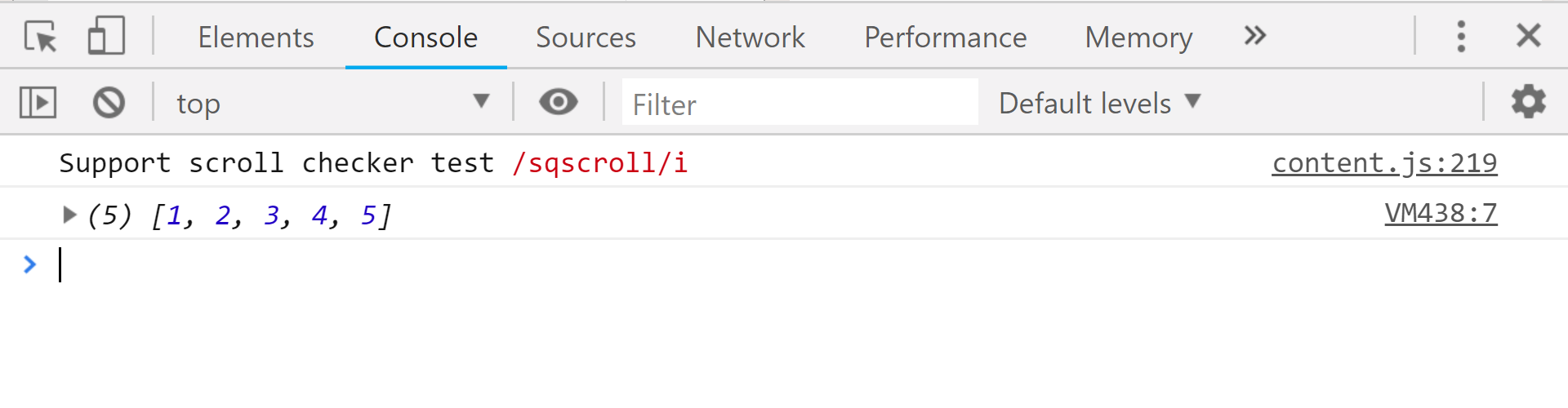We can achieve the same output with the help of the spread operator, the code will look something like this:

 `// spread operator doing the concat job ` `let arr = [1,2,3]; ` `let arr2 = [4,5]; ` ` `  `arr = [...arr,...arr2]; ` `console.log(arr); ``// [ 1, 2, 3, 4, 5 ] `

Output:Note: Though we can achieve the same result, but it is not recommended to use the spread in this particular case, as for a large data set it will work slower as when compared to the native concat() method.

Copy(like splice method)

In order to copy the content of array to another we can do something like this:

 `// copying without the spread operator ` `let arr = [``'a'``,``'b'``,``'c'``]; ` `let arr2 = arr; ` ` `  `console.log(arr2); ``// [ 'a', 'b', 'c' ] `

Output: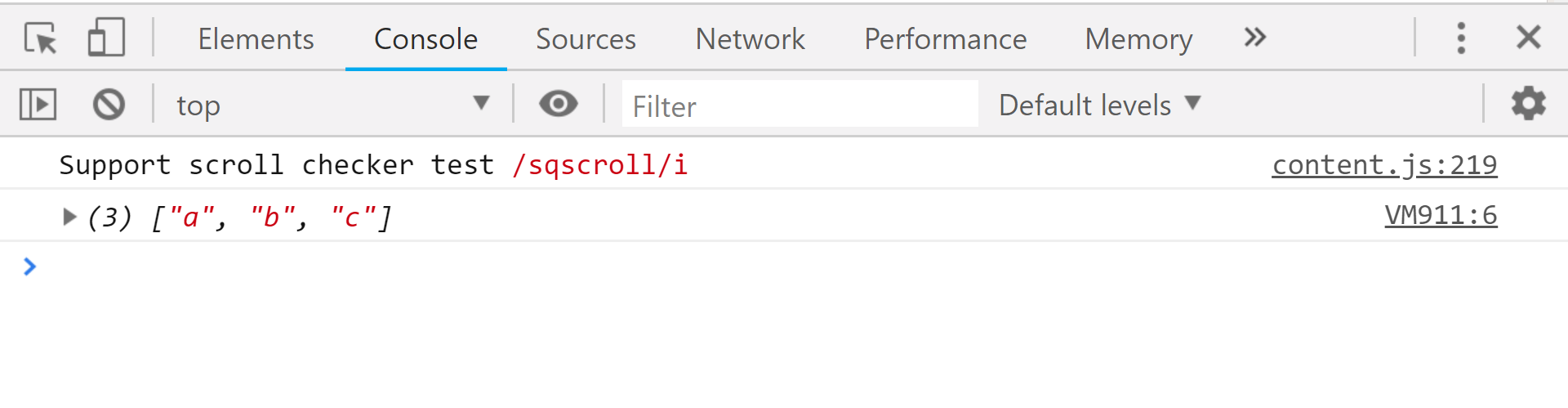The above code works fine because we can copy the contents of one array to another, but under the hood, it’s very different as when we mutate new array it will also affect the old array(the one which we copied). See the code below:

 `// changed the original array ` `let arr = [``'a'``,``'b'``,``'c'``]; ` `let arr2 = arr; ` ` `  `arr2.push(``'d'``); ` ` `  `console.log(arr2); ` `console.log(arr); ``// even affected the original array(arr)  `

Output: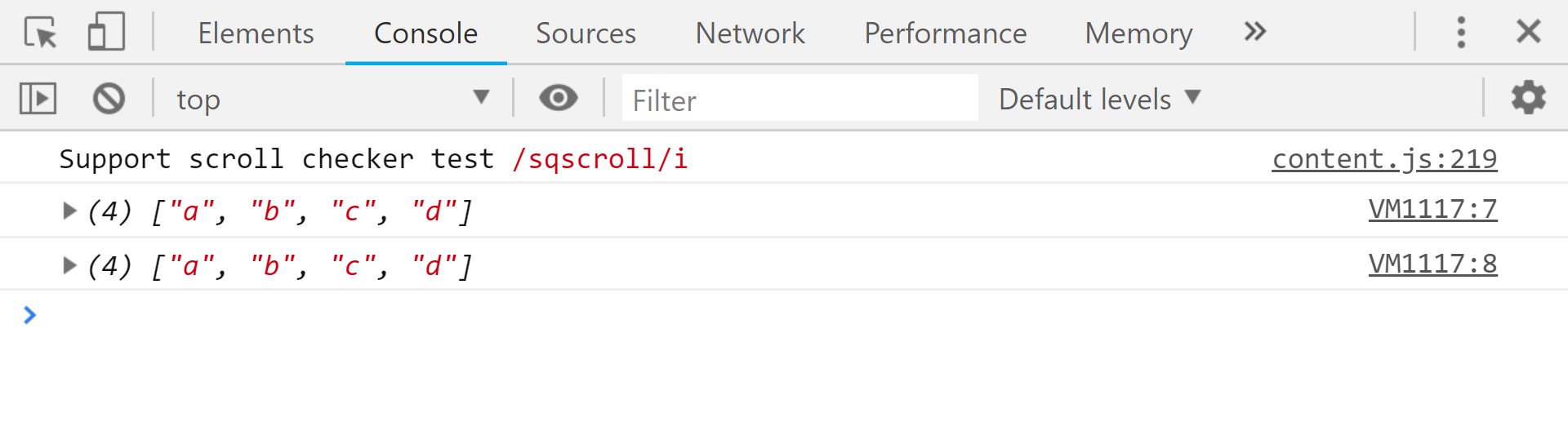In the above code we can clearly see that when we tried to insert an element inside the array, the original array is also altered which we didn’t intended and is not recommended. We can make use of the spread operator in this case, like this:

 `// spread operator for copying  ` `let arr = [``'a'``,``'b'``,``'c'``]; ` `let arr2 = [...arr]; ` ` `  `console.log(arr); ``// [ 'a', 'b', 'c' ] ` ` `  `arr2.push(``'d'``); ``//inserting an element at the end of arr2 ` ` `  `console.log(arr2); ``// [ 'a', 'b', 'c', 'd' ] ` `console.log(arr); ``// [ 'a', 'b', 'c' ] `

Output: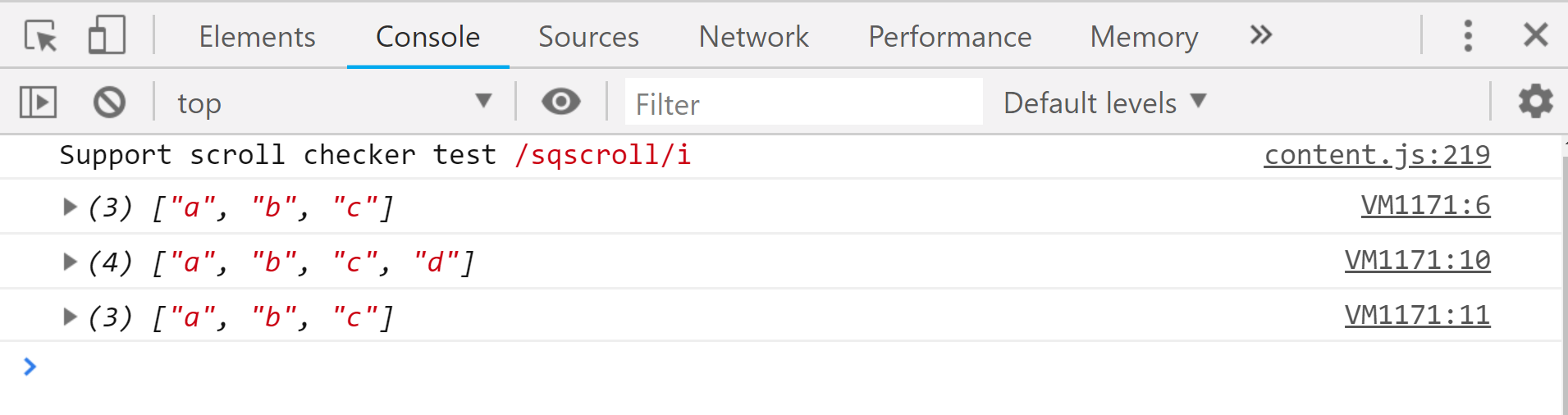By using the spread operator we made sure that the original array is not affected whenever we alter the new array.

Expand

Whenever we want to expand an array into another we do something like this:

 `// normally used expand method ` `let arr = [``'a'``,``'b'``]; ` `let arr2 = [arr,``'c'``,``'d'``]; ` ` `  `console.log(arr2); ``// [ [ 'a', 'b' ], 'c', 'd' ] `

Output: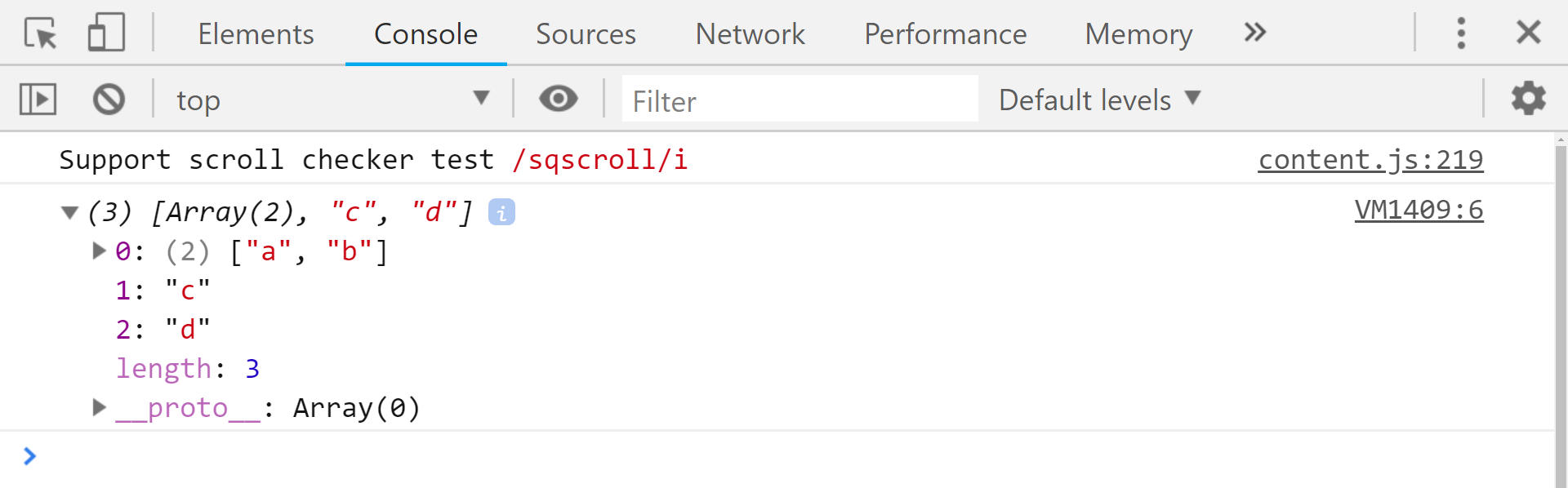Even though we get the content on one array inside the other one, but actually it is array inside another array which is definitely what we didn’t want. If we want the content to be inside a single array we can make use of the spread operator.

 `// expand using spread operator ` ` `  `let arr = [``'a'``,``'b'``]; ` `let arr2 = [...arr,``'c'``,``'d'``]; ` ` `  `console.log(arr2); ``// [ 'a', 'b', 'c', 'd' ] `

Output: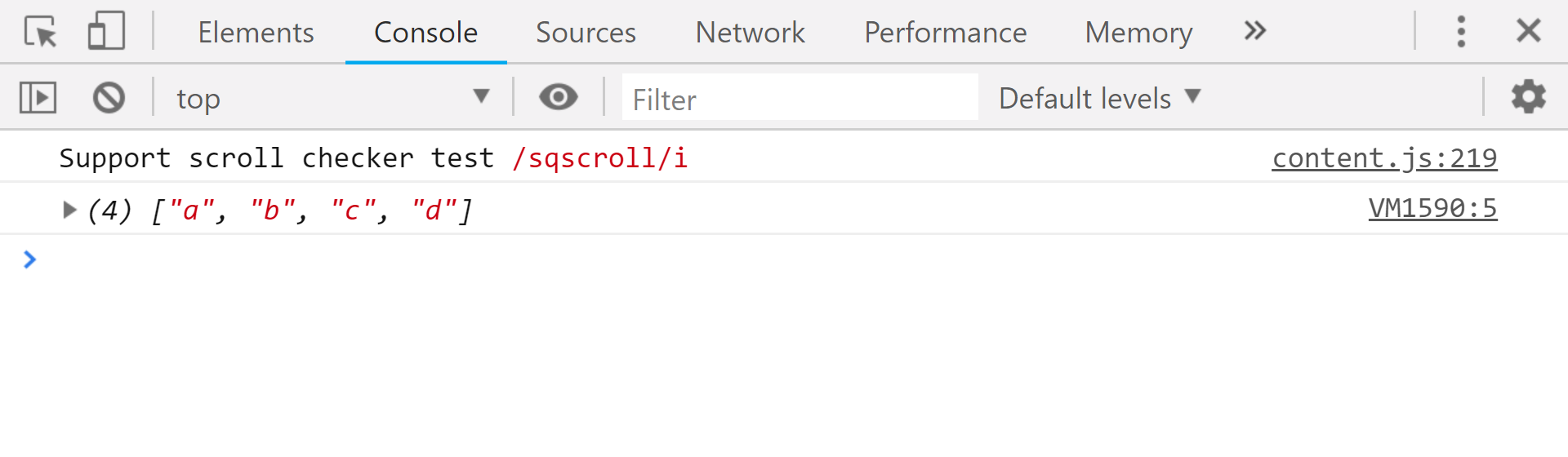Math

The Math object in javascript has different properties that we can make use of to do what we want like finding the minimum from a list of numbers, finding maximum etc. Consider the case that we want to find the minimum from a list of numbers,we will write something like this:

 `console.log(Math.min(1,2,3,-1)); ``//-1  `

Output: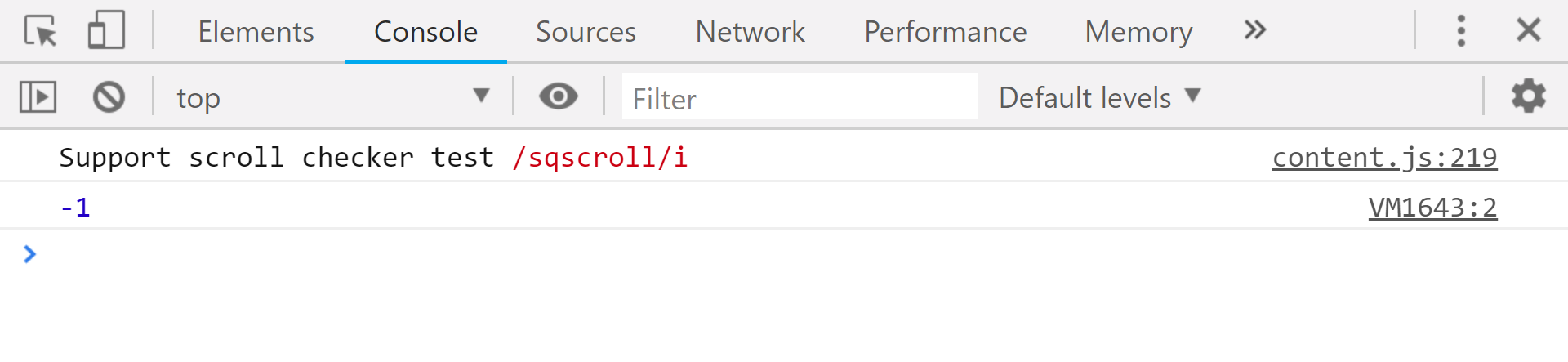Now consider that we have an array instead of a list, this above Math object method won’t work and will return NaN, like:

 `// min in an array using Math.min() ` `let arr = [1,2,3,-1]; ` `console.log(Math.min(arr)); ``//NaN `

Output: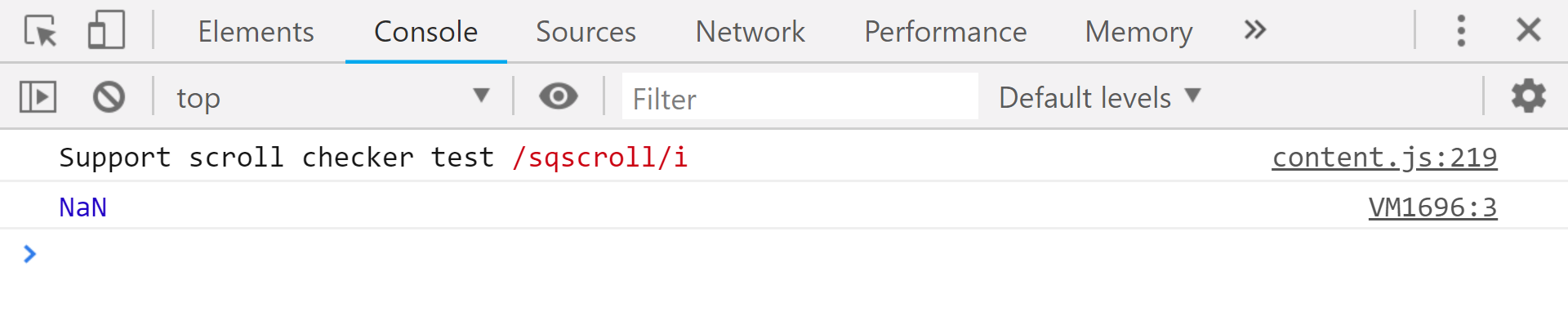When …arr is used in the function call, it “expands” an iterable object arr into the list of arguments
In order to avoid this NaN output, we make use of spread operator, like:

 `// with spread  ` `let arr = [1,2,3,-1]; ` ` `  `console.log(Math.min(...arr)); ``//-1 `

Output:Example of spread operator with objects

ES6 has added spread property to object literals in javascript. The spread operator () with objects is used to create copies of existing objects with new or updated values or to make a copy of an object with more properties. Let’s take at an example of how to use the spread operator on an object,

 `const user1 = { ` `    ``name: ``'Jen'``, ` `    ``age: 22 ` `}; ` ` `  `const clonedUser = { ...user1 }; ` `console.log(clonedUser); `

Output: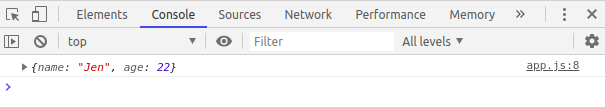Here we are spreading the user1 object. All key-value pairs of the user1 object are copied into the clonedUser object. Let’s look on another example of merging two objects using the spread operator,

 `const user1 = { ` `    ``name: ``'Jen'``, ` `    ``age: 22, ` `}; ` ` `  `const user2 = { ` `    ``name: ``"Andrew"``, ` `    ``location: ``"Philadelphia"`  `}; ` ` `  `const mergedUsers = {...user1, ...user2}; ` `console.log(mergedUsers) `

Output: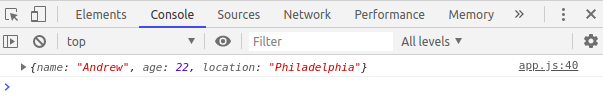mergedUsers is a copy of user1 and user2. Actually, every enumerable property on the objects will be copied to mergedUsers object. The spread operator is just a shorthand for the Object.assign() method but, they are some differences between the two.My Personal Notes arrow_drop_upCheck out this Author's contributed articles.

If you like GeeksforGeeks and would like to contribute, you can also write an article using contribute.geeksforgeeks.org or mail your article to contribute@geeksforgeeks.org. See your article appearing on the GeeksforGeeks main page and help other Geeks.

Please Improve this article if you find anything incorrect by clicking on the "Improve Article" button below.

Improved By : immukul, pranav1698

Article Tags :

9

Please write to us at contribute@geeksforgeeks.org to report any issue with the above content.